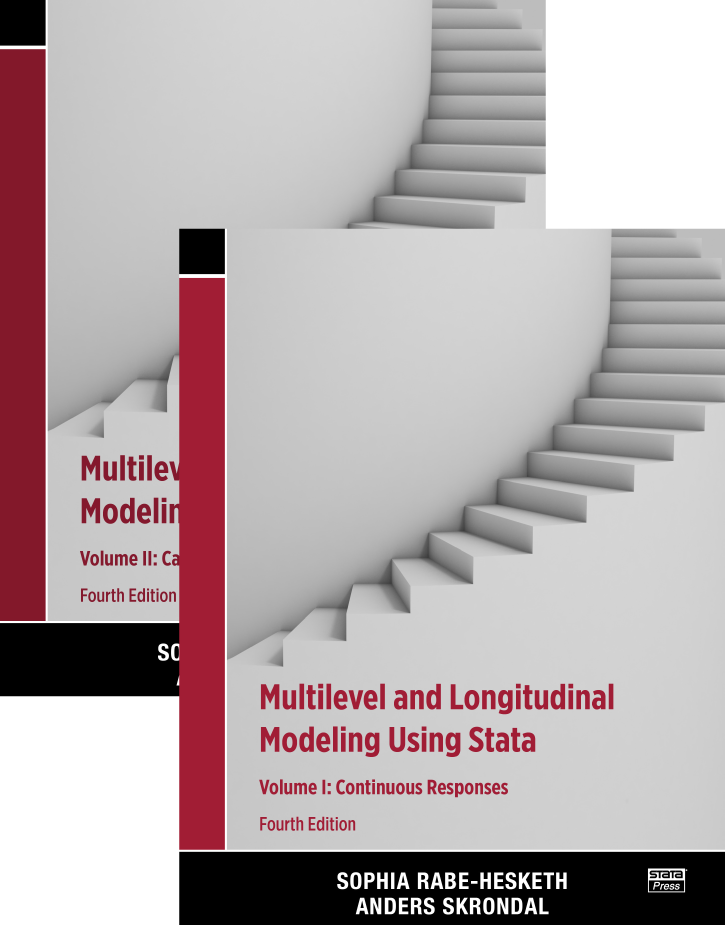# Stata Bookstore: Multilevel and Longitudinal Modeling Using Stata, 4th Edition

Sophia Rabe-Hesketh and Anders Skrondal:

Multilevel and Longitudinal Modeling Using Stata, Fourth Edition
Volume I: Continuous Responses
Volume II: Categorical Responses, Counts, and Survival

Available HERE### Comment from the Stata technical group

Multilevel and Longitudinal Modeling Using Stata, Fourth Edition, by Sophia Rabe-Hesketh and Anders Skrondal, is a complete resource for learning to model data in which observations are grouped—whether those groups are formed by a nesting structure, such as children nested in classrooms, or formed by repeated observations on the same individuals. This text introduces random-effects models, fixed-effects models, mixed-effects models, marginal models, dynamic models, and growth-curve models, all of which account for the grouped nature of these types of data. As Rabe-Hesketh and Skrondal introduce each model, they explain when the model is useful, its assumptions, how to fit and evaluate the model using Stata, and how to interpret the results. With this comprehensive coverage, researchers who need to apply multilevel models will find this book to be the perfect companion. It is also the ideal text for courses in multilevel modeling because it provides examples from a variety of disciplines as well as end-of-chapter exercises that allow students to practice newly learned material.

The book comprises two volumes. Volume I focuses on linear models for continuous outcomes, while volume II focuses on generalized linear models for binary, ordinal, count, and other types of outcomes.

Volume I begins with a review of linear regression and then builds on this review to introduce two-level models, the simplest extensions of linear regression to models for multilevel and longitudinal/panel data. Rabe-Hesketh and Skrondal introduce the random-intercept model without covariates, developing the model from principles and thereby familiarizing the reader with terminology, summarizing and relating the widely used estimating strategies, and providing historical perspective. Once the authors have established the foundation, they smoothly generalize to random-intercept models with covariates and then to a discussion of the various estimators (between, within, and random effects). The authors also discuss models with random coefficients. The text then turns to models specifically designed for longitudinal and panel data—dynamic models, marginal models, and growth-curve models. The last portion of volume I covers models with more than two levels and models with crossed random effects.

The foundation and in-depth coverage of linear-model principles provided in volume I allow for a straightforward transition to generalized linear models for noncontinuous outcomes, which are described in volume II. This second volume begins with chapters introducing multilevel and longitudinal models for binary, ordinal, nominal, and count data. Focus then turns to survival analysis, introducing multilevel models for both discrete-time survival data and continuous-time survival data. The volume concludes by extending the two-level generalized linear models introduced in previous chapters to models with three or more levels and to models with crossed random effects.

In both volumes, readers will find extensive applications of multilevel and longitudinal models. Using many datasets that appeal to a broad audience, Rabe-Hesketh and Skrondal provide worked examples in each chapter. They also show the breadth of Stata’s commands for fitting the models discussed. They demonstrate Stata’s xt suite of commands (xtregxtlogitxtpoisson, etc.), which is designed for two-level random-intercept models for longitudinal/panel data. They demonstrate the me suite of commands (mixedmelogitmepoisson, etc.), which is designed for multilevel models, including those with random coefficients and those with three or more levels. In volume 2, they discuss gllamm, a community-contributed Stata command developed by Rabe-Hesketh and Skrondal that can fit many latent-variable models, of which the generalized linear mixed-effects model is a special case.The types of models fit by the xt commands, the me commands, and gllamm sometimes overlap; when this happens, the authors highlight the differences in syntax, data organization, and output for the commands. The authors also point out the strengths and weaknesses of these commands, based on considerations such as computational speed, accuracy, available predictions, and available postestimation statistics.

The fourth edition of Multilevel and Longitudinal Modeling Using Stata has been thoroughly revised and updated. In it, you will find new material on Kenward–Roger degrees-of-freedom adjustments for small sample sizes, difference-in-differences estimation for natural experiments, instrumental-variables estimation to account for level-one endogeneity, and Bayesian estimation for crossed-effects models. In addition, you will find new discussions of meologitcmxtmixlogitmestregmenbreg, and other commands introduced in Stata since the third edition of the book.

In summary, Multilevel and Longitudinal Modeling Using Stata, Fourth Edition is the most complete, up-to-date depiction of Stata’s capacity for fitting models to multilevel and longitudinal data. Readers will also find thorough explanations of the methods and practical advice for using these techniques. This text is a great introduction for researchers and students wanting to learn about these powerful data analysis tools.